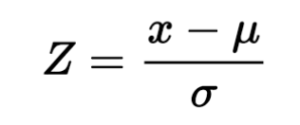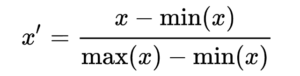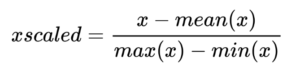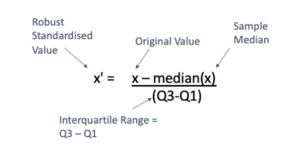Data Scaling Techniques in Machine LearningMl wizard

Data and its quality affect machine learning models and their accuracy, and the quality of the data can not be well in some types of problems which can be solved by machine learning. Here the scale of the data is also represented as the quality of the data, which needs to be expected while training the machine learning model. If the scale of the data is not normal then some techniques are applied to make it of the same scale.

Scaling is also a technique used to transform the data scale into the same scale to make it clearly understandable by machine learning algorithms and make it an easier task. This article will discuss data scaling techniques with their intuition and mathematical formulations. This will help one understand the data’s scale property and make it of the same scale if needed.

# Table of Content

• What is Data Scale?
• Standardization
• Normalization
• Min Max Scaling
• Mean Normalization
• Max Absolute Scaling
• Robust Scaling
• Key Takeaways
• Conclusion

# What is Data Scale?

The scale of the data is the range of values that every feature or column of the data has. The scale can be numerical numbers that represent data features and their values. Now here, in every case, it is not possible to have the same scale of the data ranging between the same range, and in such cases, it can cause problems like poor performance from the model.

For example, data having two columns, age and salary, where the age ranges between 0 to 100 and salary ranges between 10000 to 100,000 is having different scales, and it needs to be preprocessed before passing it to the machine learning algorithm.

Mainly two techniques are applied to achieve the same scale of the data: 1. Standardization 2. Normalization

# Standardization

Standardization is a technique where every sta point is taken, and a mathematical formula is applied to transform the particular data point.

This technique is also known as the mean centralization technique, as after applying this technique, the mean of the data points is 0, and the standard deviation of the data is 1.Image Source

Here,

Z = New Datapoint

X = data point which is to be transformed

Meu = Mena of the data

Sigma = Standard deviation of the data

As we can see in the above image, every data point referred to as “x” is taken. The mean of the data is substituted from the same and divided by the standard deviation of the data, the quantity obtained from the same is a new observation, and this will now be taken as the new data point.

# Normalization

The standardization technique is mostly preferred for scaling the data and performs well. Still, in some cases, normalization techniques are also used for transforming the scale of the data.

In this method, various techniques are used for scaling the data: 1. Min-Max Scaling 2. Mean Normalization 3. Max Absolute Scaling 4. Robust Scaling

## Min-Max Scaling

Min-max scaling is a normalization technique when we know the uppermost and lowermost limit of the data points’ values. This transformation technique converts the data points into new values ranging from 0 to 1.Image Source

Here,

X’ = New Datapoint

X = Datapoint, which is to be transformed

min(x) = Minimum value from the data

max(x) = Maximum value from the data

As we can see in the above image, the maximum and minimum value of the data is taken, and a new data point is generated, which will have a value between 0 and 1.

## Mean Normalization

Mean normalization is also a normalization technique that uses the mean of the data while transforming the data point into its new value. The mean normalization is the same as the min-max scaling techniques, which differs when it uses the mean of the data distribution instead of the data’s minimum value in the formula’s numerator.Image Source

Here,

X’ = New Datapoint

X = Datapoint, which is to be transformed

min(x) = Minimum value from the data

max(x) = Maximum value from the data

mean(X) = Mean of datapoints values

As we can see in the above image, the mean of the data is used instead of the minimum value of the data; hence, the mean normalization transforms the data points values between -1 and 1.

## Max Absolute Scaling

Max absolute scaling is a normalization technique that uses the absolute value of the maximum value of the dataset. This technique is not much used as the other methods are mostly enough to transform the data into its normal distribution.Image Source

Here,

Xscaled = New datapoint

X = Datapoints which is to be transformed

max(|x|) = Absolute value of datapoint having a maximum value from the data

## Robust Scaling

As the name suggests, robust scaling is a type of data scaling technique that is very robust to the outliers and is used in case of the data having outliers. This technique transforms the data into its normal distribution without having the effect of outliers and their values.

This technique uses the Interquartile range and median of the data, which makes it robust to the outliers.Image Source

As we can see in the above image, the technique uses the median in its numerator and the IQR in its denominator, making it robust to the outliers as the median and IQR do not have any relationship with outliers and their value in any way.

# Key Takeaways

• The data points and their value should be appropriately scaled before feeding them to the machine learning algorithms to avoid poor performance.
• The standardization techniques are the most frequently used technique that transforms the data to have zero mean and one standard deviation after transformation.
• The min-max scaling transforms the data between 0 to 1 by using maximum and minimum values of the data.
• The mean normalization technique transforms the data between -1 to 1 by using the mean of the data.
• The maximum absolute technique is not much used, but sometimes it may help with data that does not fit with other cling techniques.
• The robust scaling would be used in case of outliers present where it transformers the data without any effect of outliers and their values.

# Conclusion

In this article, we discussed data scaling and its techniques to transform the data into the same scale to make the model training more straightforward and efficient. This will help one to understand the scale f the data and apply an appropriate technique to bring the scale of the data into the correct format.